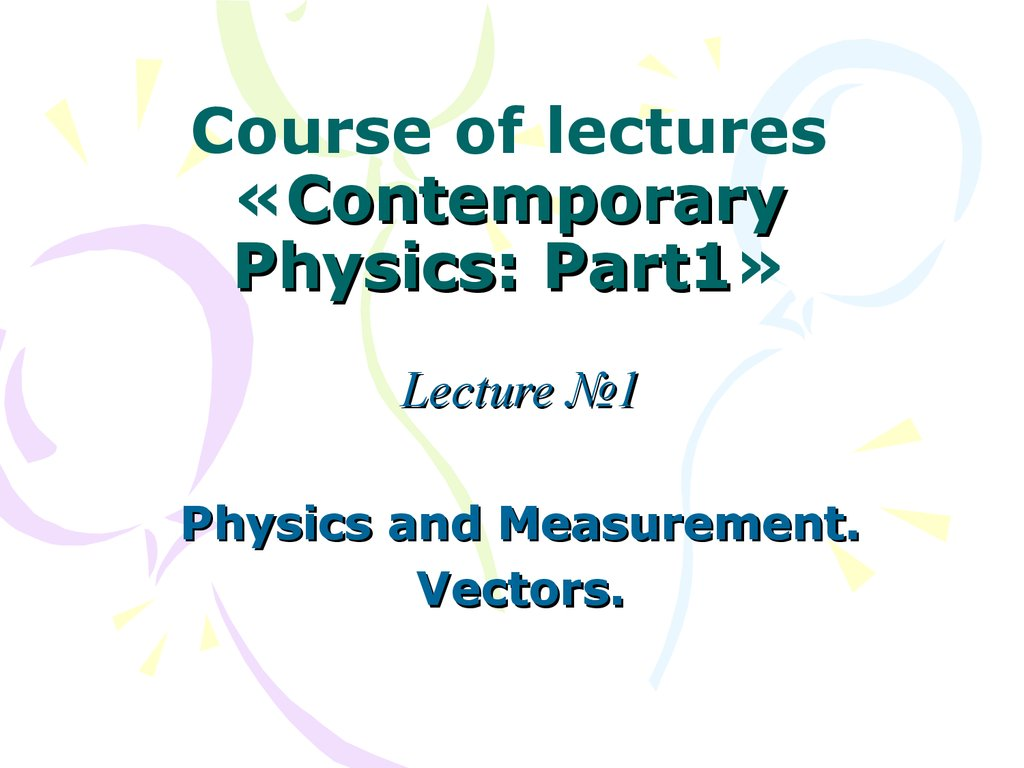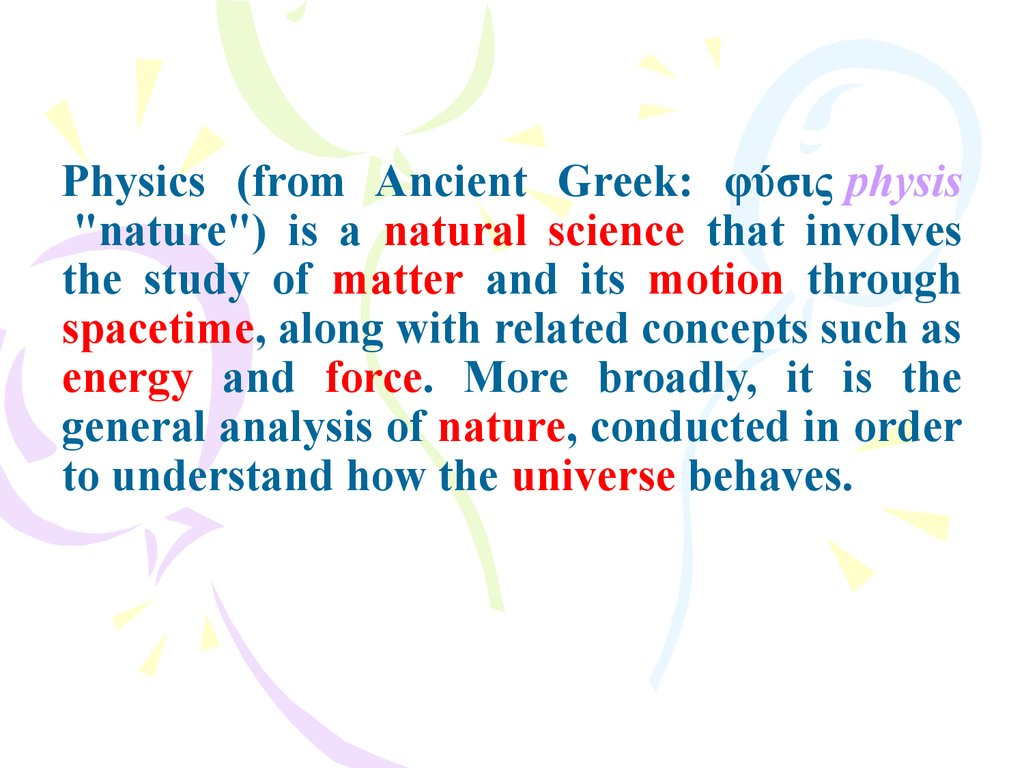# Physics and Measurement. Vectors. Course of lectures «Contemporary Physics: Part1». Lecture 1

## 1. Course of lectures «Contemporary Physics: Part1»

Part1
Lecture №1
Physics and Measurement.
Vectors.

## 2.

Various examples of
physical phenomena

## 3.

Physics (from Ancient Greek: φύσις physis
"nature") is a natural science that involves
the study of matter and its motion through
spacetime, along with related concepts such as
energy and force. More broadly, it is the
general analysis of nature, conducted in order
to understand how the universe behaves.

## 5.

The basic domains of physics

## 6.

History of physics
Aristotle (384–322 BCE)
Galileo Galilei (1564–1642)

## 7.

Isaac Newton (1643–1727)

## 8.

Clausius (1822-1888)
Albert Einstein (1879–1955)

## 9.

Base units are: kg, m, s, A, K, mol and cd.
In Si system this units have independent dimension.

arithmetic mean

## 15.

Absolute error and
relative error

## 16.

Standard deviation

## 18.

Frame of reference
A frame of reference in
physics, may refer to a
coodinate system or set of
axes within which to
measure
the
position,
orientation, and other
properties of objects in it,
or it may refer to an
observational
reference
frame tied to the state of
motion of an observer. It
may also refer to both an
observational
reference
frame and an attached
coordinate system, as a
unit.

## 23.

Dot product
The dot product of two vectors a and b (sometimes called the
inner product, or, since its result is a scalar, the scalar product) is
denoted by a ∙ b and is defined as:
where θ is the measure of the angle between a and b (see
trigonometric function for an explanation of cosine).
Geometrically, this means that a and b are drawn with a common
start point and then the length of a is multiplied with the length of
that component of b that points in the same direction as a.
The dot product can also be defined as the sum of the products of the
components of each vector as

## 24.

Cross product
The cross product (also called the vector product or outer product)
is only meaningful in three dimensions. The cross product differs from
the dot product primarily in that the result of the cross product of two
vectors is a vector. The cross product, denoted a × b, is a vector
perpendicular to both a and b and is defined as:

## 26.

Divergence
Application in Cartesian coordinates
Let x, y, z be a system of Cartesian coordinates on a 3-dimensional
Euclidean space, and let i, j, k be the corresponding basis of
unit vectors.
The divergence of a continuously differentiable vector field F = U i
+ V j + W k is equal to the scalar-valued function:

## 27.

Curl
In vector calculus, the curl (or rotor) is a vector operator that
describes the infinitesimal rotation of a 3-dimensional vector
field. At every point in the field, the curl is represented by a
vector. The attributes of this vector (length and direction)
characterize the rotation at that point.

## 28.

Using vectors in physics